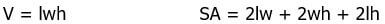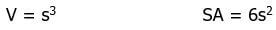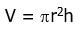## Geometry Formulas

Geometry is a subdivision of the subject mathematics that is all about shape, size, the properties of space and relative position of figures. It was also predominant many cultures of earlier times and has always been a practical way of calculating lengths, areas, and volumes using geometry formulas.

Geometry is divided into two types: Plane Geometry and Solid Geometry. Plane Geometry is for shapes such as triangles, circles, rectangles, square, geometry formulas and much more. Solid Geometry deals with calculating the perimeter, length, area, and volume of different geometric figures and shapes. It is also used for calculating the arc length and radius, etc.

The main thing every student needs to know about this subject are the Geometry Formulas. Geometry Formulas are crucial elements to calculate the perimeter, length, area, and volume of geometric shapes and figures. There are loads of geometric formulas that are concerned with height, length, width, radius, perimeter, area, volume or surface area.

Many of the geometric formulas are quite complicated and there are some you must have hardly ever seen. However, the basic geometry formulas are widely used in our daily life to calculate the length, space and so on.

## Basic Geometry Formulas

PerimeteoSquar4Perimeter o fa Square = P = 4a

Where a = Length of the sides of a Square

PerimeteoRectangl2(l+bPerimeter of aRectangle = P = 2(l+b)

Where, l = Length ; b = Breadth

AreoSquaraArea of a Square = A = a2

Where a = Length of the sides of a Square

AreoRectangll×Area of a Rectangle = A = l×b

Where, l = Length ; b = Breadth

AreoTrianglb× hArea of a Triangle = A = b×h2

Where b = base of the triangle; h = height of the triangle

AreoTrapezoi(b1+b2hArea of a Trapezoid = A = (b1+b2) h2

Where, b1b1 & b2b2 are the bases of the Trapezoid; h = height of the Trapezoid

AreoCirclπ×rArea of a Circle=A=π×r2

CircumferencoCircle=A=2πCircumference of a Circle=A=2πr

Where, r = Radius of the Circle

SurfacAreoCube=S=6a2 SurfacAreoCube=S=6a2

Where, a = Length of the sides of a Cube

SurfacAreoCylinder=S=2πrh SurfacAreoCylinder=S=2πrh

VolumoCylinder=V=πr2h VolumoCylinder=V=πr2h

Where, r = Radius of the base of the Cylinder; h = Height of the Cylinder

SurfacAreoCone=S=πr(r+h2+r2−−−−−−√) SurfacAreoCone=S=πr(r+h2+r2)

VolumoCone=V=πr2h VolumoCone=V=πr2h

Where, r = Radius of the base of the Cone, h = Height of the Cone

SurfacAreoSphere=S=4πr2 SurfacAreoSphere=S=4πr2

VolumoSphere=V=43πr3 VolumoSphere=V=43πr3

Where, r = Radius of the Sphere

## Angles and Lines

A right angle consists of 90 degrees.  A straight line is made up of 180 degrees.Take a look at this diagram; ADC is a straight line.  Make sure that you don’t confuse “straight” lines with “horizontal” lines.  Any line that does not bend is “straight,” irrespective of the direction.  Also, a “horizontal” line is parallel to the top or bottom of the page, parallel to the distant horizon.  Line ADC is “straight” but not “horizontal.”

Notice that the “straightness” is one of the very few things we can assume on the GRE.  It seems like there’s no bend in the line, then there’s no bend: i.e. if it looks straight, it is straight.  On the contrary, one cannot assume perpendicularity.

If two lines intersect, the sum of the resulting four angles equals 360°.  In addition, the angles opposite each need to be equal as they meet at just a vertex, so they are called “vertical” angles.If an angle AED equals 40°, then we will know the measures of the other three angles: angle BEC would have to equal AED, so that would also be 40°, and the other two angles would have to be the supplements of 40°.  The supplement of an angle is 180° minus the angle.  Thus, angle AEB = angle DEC = 140°; two angles that add up to 180° are supplementary.  This way, all four angles add up to 360°.

There are a lot more angles when two parallel lines are intersected by a third line.  We get eight angles: four “big” angles and four “small” angles.  There are a number of technical names (“alternate interior angles”) that we don’t have to know for GRE geometry.  We just have to know that every big angle is equal, every small angle is equal, and any big angle and any small angle are supplementary. Lines are an important part of Geometry Formulas.

## All Triangles – Geometry Formulas

A triangle is a shape that has three line segment sides.Here are some facts about all triangles:

In any triangle, the sum of the three angles is 180°.

That’s the great idea that Mr. Euclid (c. 300 BCE), the great geometer, first came up with.  Take a look at another crucial geometry equation:The letter b stands for the base: what’s the base of a triangle? Interestingly, any of the three sides can be the base.  Similarly, the letter h is height: by “height,” we mean the length of a segment known as an altitude.  An altitude is a line that passes through a vertex and is perpendicular to the side we are considering the base.  So, for any triangle, we will have three different b & h combinations that would allow us to calculate the area.Of course, the GRE will not give you all that information, three side lengths, and three altitudes, in the course of a geometry problem.  If a GRE geometry question is about the area of the triangle, it will also give a way to find at least one base and the corresponding height.  Don’t forget that the altitude divides the triangle into two little right triangles, so the Pythagorean Theorem (below) may be involved in finding some of the necessary lengths.

## Isosceles Triangles – Geometry Formulas

The geometry rule about this last fact is about one elite category of triangles: the isosceles triangles.  These are triangles with two equal sides.The angle between the two equal sides may be

(a) an acute angle (less than 90°), as in triangle ABD

(b) a right angle, as in triangle DEF, or

(c) an obtuse angle (greater than 90°) as in triangle KLM

If two sides are equal, then we know the opposite angles are also equal: in triangle ABC, angle A = angle C; in triangle DEF, angle E = angle F; and in triangle KLM, angle K = angle M.  For that matter, Mr. Euclid figured out that this geometry rule works both ways: if we are told two sides are equal, then we can conclude that two angles are equal, and if we are told two angles are equal, we know two sides are equal.

The line that goes down the middle of an isosceles triangle is special:As we know, JKL and PQR are isosceles triangles, so the midline has many special properties:

(a) it is perpendicular to the base: angle KML = angle QSR = 90°

(b) its lower point bisects the base: JM = ML and PS = SR

(c) it bisects the upper angle: angle JKM = angle MKL, and angle PQS = angle SQR

These are some great geometry formulas and equations that can be associated with isosceles triangles.

## Right Triangles – Geometry Formulas

If there are right triangles with two sides touching the right angle, they are called legs.  The longest side, which is always opposite the right angle, is called the hypotenuse.

The one important rule is applicable to all right triangles is theorem named for Mr. Pythagoras (570 – 495 BCE), who is considered to be the first mathematician.Take a look at the figure and you’ll notice that the side we call “c,” the side alone on one side of the equation, has to be the hypotenuse.  Also, we can use this formula only when we know a triangle is a right triangle, and it’s futile to just assume anything.  If there’s the litter perpendicular square, then we can conclude it’s a right triangle and we can apply this geometry equation.

Of course, many of these sides of a right triangle can be decimals, but only in special cases, for all three sides to be integers.  These are sets of three integers that satisfy the Pythagorean Theorem.  The most common is {3, 4, 5} and its multiples, but other good ones to recognize are {5, 12, 13}, {8, 15, 17}, and {7, 24, 25}.

There are two important triangles we need to understand for GRE geometry.

The first is the 30-60-90 triangle:The other triangle is the 45-45-90 triangle, also known as the Isosceles Right Triangle.## Higher Polygons

A polygon is basically a closed figure that has three or more line-segment sides. Here’s a chart for the names:The sum of all the angles of the polygon is given by sum = (n – 2)(180°) for any n-sided polygon.  If a triangle has a sum of 180°, then any quadrilateral has a sum of 360°; any pentagon has a sum of 540°, and so on.

Another crucial idea is that of a regular polygon.  This is tricky word as generally, “regular” means ordinary, commonplace. But in geometry, it almost has the opposite meaning.  The regular polygons are one of the elite and symmetrical shapes, having equal sides and all equal angles.  The “regular triangle” is the equilateral triangle, and the “regular quadrilateral” is the square.   In a regular polygon, we can divide this by the number of angles to arrive at individual angle measures as we can compute the sum of the angles.Of course, a regular octagon is the stop sign shape in many places around the world.

As seen above, quadrilaterals consist of two diagonals.  Pentagons have five, and higher polygons have much more. The diagonals of the regular pentagon trace out the standard five-pointed star, such as the stars on the flag of the United States of America.

## Three-Dimensional Shapes

rectangular solid is primarily a box-shape with six faces that are rectangles, but all the faces intersect at only right angles. Let’s assume that it has a height of h, a length of l, and width of w.  Then the calculation of volume and surface area would be:The surface area is nothing but the sum of the areas of the six rectangular faces.

In case, all the lengths of a rectangle solid are equal, then it’s called a cube.  A cube includes six congruent square faces which meet at right angles to each other. Let’s say of the edge length is s, thenAll kinds of 3D shapes that include a circle were discovered by Archimedes (287 – 212 BCE), including the sphere and the cylinder.  The volume of a cylinder can also be considered as the base times height, where the “base” is the area of the circular base.The sphere is the most symmetrical 3D shape.

V = 4/3πr^3

SA = 4πr^2

## Coordinate Geometry – Geometry Formulas

A line in the coordinate plane can be described in a few ways: the most widely accepted is the slope-intercept form:

y = mx + bThe b is the y-intercept, it’s a place where the line intersects the y-axis.  The m is the slope.  Slope indicates how tilted a line is.  A horizontal line has a slope of zero.  A vertical line has an infinite slope.  A 45° line has a slope of m = 1.  To find the slope between two points A & B, draw a slope triangle:

When we do a rough sketch, then the slope is just a rise over run. There are certainly a few ways to think about any slope.

A slope of m = +3 means any of the following:
(a) to the right one unit, up 3 units

(b) any multiple of (a) (e.g. right 7 units, up 21 units)

(c) to the left one unit, down 3 units

(d) any multiple of (b) (e.g. left 5 units, down 15 units)

A slope of m = – 5/3 means any of the following:

(a) to the right 1 unit, down 5/3 of a unit

(b) to the right 3 units, down 5 units

(c) any multiple of (b) (e.g.  right 9 units, down 15 units)

(d) to the left 1 unit, up 5/3 of a unit

(e) to the left 3 units, up 5 units

(f) any multiple of (e) (e.g.  left 18 units, up 30 units)

The geometry rules concerning slope are very important to remember.

To find the y-intercept, set x equal to 0 and solve for y.

To find the x-intercept, set y equal to 0 and solve for x.

That was all about Geometry formulas, folks.

You may also like:

In focus: 3D geometry

Euclidean geometry

7 Tips to approach problems in coordinate geometry

## What will your All India Rank be?

Compete with lakhs of students across India on tests created by the best teachers in India.

+91
No thanks.

## Request a Free 60 minute counselling session at your home

Please enter a valid phone number
•7,829,648

Happy Students
•358,177,393

Questions Attempted
•3,028,498

Tests Taken
•3,020,367